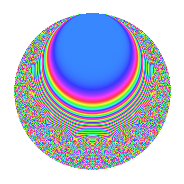# Properties

 Label 354.6.aLevel 354 Weight 6 Character orbit a Rep. character $$\chi_{354}(1,\cdot)$$ Character field $$\Q$$ Dimension 48 Newform subspaces 9 Sturm bound 360 Trace bound 5

# Related objects

## Defining parameters

 Level: $$N$$ = $$354 = 2 \cdot 3 \cdot 59$$ Weight: $$k$$ = $$6$$ Character orbit: $$[\chi]$$ = 354.a (trivial) Character field: $$\Q$$ Newform subspaces: $$9$$ Sturm bound: $$360$$ Trace bound: $$5$$ Distinguishing $$T_p$$: $$5$$

## Dimensions

The following table gives the dimensions of various subspaces of $$M_{6}(\Gamma_0(354))$$.

Total New Old
Modular forms 304 48 256
Cusp forms 296 48 248
Eisenstein series 8 0 8

The following table gives the dimensions of the cuspidal new subspaces with specified eigenvalues for the Atkin-Lehner operators and the Fricke involution.

$$2$$$$3$$$$59$$FrickeDim.
$$+$$$$+$$$$+$$$$+$$$$6$$
$$+$$$$+$$$$-$$$$-$$$$6$$
$$+$$$$-$$$$+$$$$-$$$$8$$
$$+$$$$-$$$$-$$$$+$$$$5$$
$$-$$$$+$$$$+$$$$-$$$$6$$
$$-$$$$+$$$$-$$$$+$$$$5$$
$$-$$$$-$$$$+$$$$+$$$$4$$
$$-$$$$-$$$$-$$$$-$$$$8$$
Plus space$$+$$$$20$$
Minus space$$-$$$$28$$

## Trace form

 $$48q - 8q^{2} + 18q^{3} + 768q^{4} + 132q^{5} - 276q^{7} - 128q^{8} + 3888q^{9} + O(q^{10})$$ $$48q - 8q^{2} + 18q^{3} + 768q^{4} + 132q^{5} - 276q^{7} - 128q^{8} + 3888q^{9} + 624q^{10} - 632q^{11} + 288q^{12} + 1448q^{13} - 792q^{15} + 12288q^{16} + 3972q^{17} - 648q^{18} + 1816q^{19} + 2112q^{20} + 3168q^{21} + 3824q^{22} - 400q^{23} + 26928q^{25} + 7024q^{26} + 1458q^{27} - 4416q^{28} + 1332q^{29} - 8352q^{30} - 7492q^{31} - 2048q^{32} - 720q^{33} - 10288q^{34} + 41000q^{35} + 62208q^{36} + 21136q^{37} - 14048q^{38} - 684q^{39} + 9984q^{40} - 28172q^{41} + 11788q^{43} - 10112q^{44} + 10692q^{45} + 3456q^{46} + 6272q^{47} + 4608q^{48} + 113700q^{49} + 38216q^{50} - 15444q^{51} + 23168q^{52} + 8852q^{53} - 103128q^{55} - 11448q^{57} + 304q^{58} - 12672q^{60} + 44800q^{61} - 63424q^{62} - 22356q^{63} + 196608q^{64} - 132912q^{65} + 23040q^{66} + 1412q^{67} + 63552q^{68} + 70488q^{69} + 45984q^{70} - 135200q^{71} - 10368q^{72} - 65528q^{73} - 117232q^{74} + 59886q^{75} + 29056q^{76} + 249296q^{77} + 24336q^{78} + 147604q^{79} + 33792q^{80} + 314928q^{81} - 169360q^{82} + 206720q^{83} + 50688q^{84} + 259968q^{85} + 157152q^{86} - 82728q^{87} + 61184q^{88} + 374524q^{89} + 50544q^{90} + 241072q^{91} - 6400q^{92} + 18972q^{93} + 100128q^{94} + 639464q^{95} + 52528q^{97} - 7880q^{98} - 51192q^{99} + O(q^{100})$$

## Decomposition of $$S_{6}^{\mathrm{new}}(\Gamma_0(354))$$ into newform subspaces

Label Dim. $$A$$ Field CM Traces A-L signs $q$-expansion
$$a_2$$ $$a_3$$ $$a_5$$ $$a_7$$ 2 3 59
354.6.a.a $$1$$ $$56.776$$ $$\Q$$ None $$4$$ $$-9$$ $$10$$ $$144$$ $$-$$ $$+$$ $$+$$ $$q+4q^{2}-9q^{3}+2^{4}q^{4}+10q^{5}-6^{2}q^{6}+\cdots$$
354.6.a.b $$4$$ $$56.776$$ 4.4.32832.1 None $$16$$ $$36$$ $$-104$$ $$-162$$ $$-$$ $$-$$ $$+$$ $$q+4q^{2}+9q^{3}+2^{4}q^{4}+(-5^{2}+2\beta _{1}+\cdots)q^{5}+\cdots$$
354.6.a.c $$5$$ $$56.776$$ $$\mathbb{Q}[x]/(x^{5} - \cdots)$$ None $$-20$$ $$45$$ $$-10$$ $$-162$$ $$+$$ $$-$$ $$-$$ $$q-4q^{2}+9q^{3}+2^{4}q^{4}+(-2+\beta _{1}+\cdots)q^{5}+\cdots$$
354.6.a.d $$5$$ $$56.776$$ $$\mathbb{Q}[x]/(x^{5} - \cdots)$$ None $$20$$ $$-45$$ $$-24$$ $$-103$$ $$-$$ $$+$$ $$-$$ $$q+4q^{2}-9q^{3}+2^{4}q^{4}+(-5+\beta _{3}+\cdots)q^{5}+\cdots$$
354.6.a.e $$5$$ $$56.776$$ $$\mathbb{Q}[x]/(x^{5} - \cdots)$$ None $$20$$ $$-45$$ $$166$$ $$-198$$ $$-$$ $$+$$ $$+$$ $$q+4q^{2}-9q^{3}+2^{4}q^{4}+(33-\beta _{2})q^{5}+\cdots$$
354.6.a.f $$6$$ $$56.776$$ $$\mathbb{Q}[x]/(x^{6} - \cdots)$$ None $$-24$$ $$-54$$ $$-46$$ $$-103$$ $$+$$ $$+$$ $$+$$ $$q-4q^{2}-9q^{3}+2^{4}q^{4}+(-8+\beta _{5})q^{5}+\cdots$$
354.6.a.g $$6$$ $$56.776$$ $$\mathbb{Q}[x]/(x^{6} - \cdots)$$ None $$-24$$ $$-54$$ $$4$$ $$-54$$ $$+$$ $$+$$ $$-$$ $$q-4q^{2}-9q^{3}+2^{4}q^{4}+(1-\beta _{5})q^{5}+\cdots$$
354.6.a.h $$8$$ $$56.776$$ $$\mathbb{Q}[x]/(x^{8} - \cdots)$$ None $$-32$$ $$72$$ $$40$$ $$181$$ $$+$$ $$-$$ $$+$$ $$q-4q^{2}+9q^{3}+2^{4}q^{4}+(5+\beta _{1})q^{5}+\cdots$$
354.6.a.i $$8$$ $$56.776$$ $$\mathbb{Q}[x]/(x^{8} - \cdots)$$ None $$32$$ $$72$$ $$96$$ $$181$$ $$-$$ $$-$$ $$-$$ $$q+4q^{2}+9q^{3}+2^{4}q^{4}+(12-\beta _{1})q^{5}+\cdots$$

## Decomposition of $$S_{6}^{\mathrm{old}}(\Gamma_0(354))$$ into lower level spaces

$$S_{6}^{\mathrm{old}}(\Gamma_0(354)) \cong$$ $$S_{6}^{\mathrm{new}}(\Gamma_0(3))$$$$^{\oplus 4}$$$$\oplus$$$$S_{6}^{\mathrm{new}}(\Gamma_0(6))$$$$^{\oplus 2}$$$$\oplus$$$$S_{6}^{\mathrm{new}}(\Gamma_0(59))$$$$^{\oplus 4}$$$$\oplus$$$$S_{6}^{\mathrm{new}}(\Gamma_0(118))$$$$^{\oplus 2}$$$$\oplus$$$$S_{6}^{\mathrm{new}}(\Gamma_0(177))$$$$^{\oplus 2}$$